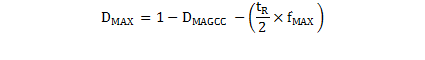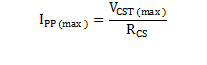SLUSBL5A February   2015  – June 2019

PRODUCTION DATA.

1. Features
2. Applications
3. Description
1.     Device Images
4. Revision History
5. Pin Configuration and Functions
6. Specifications
7. Detailed Description
1. 7.1 Overview
2. 7.2 Functional Block Diagram
3. 7.3 Feature Description
4. 7.4 Device Functional Modes
8. Application and Implementation
1. 8.1 Application Information
2. 8.2 Typical Application
3. 8.3 Do's and Don'ts
9. Power Supply Recommendations
10. 10Layout
11. 11Device and Documentation Support
1. 11.1 Device Support
1. 11.1.1 Development Support
2. 11.1.2 Device Nomenclature
2. 11.2 Documentation Support
3. 11.3 Receiving Notification of Documentation Updates
4. 11.4 Community Resources
5. 11.5 Trademarks
6. 11.6 Electrostatic Discharge Caution
7. 11.7 Glossary
12. 12Mechanical, Packaging, and Orderable Information

• D|7

#### 8.2.2.3 Transformer Turns Ratio, Inductance, Primary-Peak Current

The maximum primary-to-secondary turns ratio can be determined by the target maximum switching frequency at full load, the minimum input capacitor bulk voltage, and the estimated DCM resonant time.

First, determine the maximum duty cycle of the MOSFET based on the target maximum switching frequency, fMAX, the secondary conduction duty cycle, DMAGCC, and the DCM resonant period, tR. For tR, assume 2 µs (500-kHz resonant frequency), if you do not have an estimate from experience or previous designs. For the transition mode operation limit, the time interval from the end of the secondary current conduction to the first resonant valley of the VDS voltage is ½ of the DCM resonant period, or 1 µs assuming 500 kHz. Actual designs vary. DMAX can be determined using Equation 10.

Equation 10.DMAGCC is defined as the secondary diode conduction duty cycle during constant current, CC, operation. In the UCC28730, it is fixed internally at 0.432. Once DMAX is known, the ideal turns ratio of the primary-to-secondary windings can be determined with the equation below. The total voltage on the secondary winding needs to be determined, which is the total of VOCV, the secondary rectifier drop VF, and cable compensation voltage VOCBC, if used. For 5-V USB charger applications, for example, a turns ratio in the range of 13 to 15 is typically used.

Equation 11.The actual turns ratio depends on the actual number of turns on each of the transformer windings. Choosing NPS > NPS(ideal) results in an output power limit lower than (VOCV x IOCC) when operating at VIN(min), and line-frequency ripple may appear on VOUT. Choosing NPS < NPS(ideal) allows full-power regulation down to VIN(min), but increases conduction losses and the reverse voltage stress on the output rectifier.

Once the actual turns ratio is determined from a detailed transformer design, use this ratio for the following parameter calculations.

The UCC28730 constant-current regulation is achieved by maintaining a maximum DMAGCC duty cycle of 0.432 at the maximum primary current setting. The transformer turns ratio and constant-current regulating factor determine the current-sense resistor, RCS, for a regulated constant-current target, IOCC. Actual implementation of RCS may consist of multiple parallel resistors to meet power rating and accuracy requirements.

Because not all of the energy stored in the transformer is transferred to the secondary output, a transformer efficiency term, ηXFMR, is used to account for the core and winding loss ratio, leakage inductance loss ratio, and primary bias power ratio with respect to the rated output power. At full load, an overall transformer efficiency estimate of 0.91, for example, includes ~3% leakage inductance loss, ~5% core and winding loss, and ~1% bias power. Actual loss ratios may vary from this example.

Equation 12.The primary-transformer inductance can be calculated using the standard energy storage equation for flyback transformers. Primary current, maximum switching frequency and output and transformer power losses are included in the equation below.

Initially, determine the transformer peak primary current, IPP(max).

Peak-primary current is simply the maximum current-sense threshold divided by the current-sense resistance.

Equation 13.Then, calculate the primary inductance of the transformer, LP.

Equation 14.The auxiliary winding to secondary winding turns ratio, NAS, is determined by the lowest target operating output voltage in constant current regulation, the VDD turn-off threshold of the UCC28730, and the forward diode drops in the respective winding networks.

Equation 15.There is additional energy supplied to VDD from the transformer leakage inductance energy which may allow a lower turns ratio to be used in many designs.